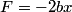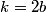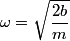## Solution to 1986 Problem 60

 The force associated with this potential is\begin{align*}F = - 2 b x\end{align*}So, the spring constant is$k = 2 b$ and the angular frequency of the oscillations is:\begin{align*}\omega = \sqrt{\frac{2b}{m}}\end{align*}This depends only on$b$ and$m$, hence answer (C) is correct.